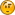January 29, 2020, 09:11:19 am

## News:

GP Bikes beta16b available!## Tyres Physics (pacejka magic formula)

Started by Manu, April 16, 2018, 10:28:42 pm

#### Manu

#####April 16, 2018, 10:28:42 pmLast Edit: April 19, 2018, 03:25:52 pm by Manu

MAGIC FORMULA

Fz0 = Nominal normal force
p_Cx1 = Shape factor Cfx for longitudinal force
p_Dx1 = Longitudinal friction Mux at Fznom
p_Dx2 = Variation of friction Mux with load
p_Ex1 = Longitudinal curvature Efx at Fznom
p_Ex2 = Variation of curvature Efx with load
p_Ex3 = Variation of curvature Efx with load squared
p_Ex4 = Factor in curvature Efx while driving
p_Kx1 = Longitudinal slip stiffness Kfx/Fz at Fznom
p_Kx2 = Variation of slip stiffness Kfx/Fz with load
p_Kx3 = Exponent in slip stiffness Kfx/Fz with load
r_Bx1 = Slope factor for combined slip Fx reduction
r_Bx2 = Variation of slope Fx reduction with kappa
r_Bx3 = Influence of camber on stiffness for Fx combined
r_Cx1 = Shape factor for combined slip Fx reduction
p_Cy1 = Shape factor Cfy for lateral forces
p_Cy2 = Shape factor Cfc for camber forces
p_Dy1 = Lateral friction Muy
p_Dy2 = Variation of friction Muy with load
p_Dy3 = Variation of friction Muy with squared camber
p_Ey1 = Lateral curvature Efy at Fznom
p_Ey2 = Variation of curvature Efy with load
p_Ey3 = Zero order camber dependency of curvature Efy
p_Ey4 = Variation of curvature Efy with camber
p_Ey5 = Camber curvature Efc
p_Ky1 = Maximum value of stiffness Kfy/Fznom
p_Ky2 = Load at which Kfy reaches maximum value
p_Ky3 = Variation of Kfy/Fznom with camber
p_Ky4 = Peak stiffness variation with camber squared
p_Ky5 = Lateral stiffness depedency with camber
p_Ky6 = Camber stiffness factor
p_Ky7 = Load dependency of camber stiffness factor
r_By1 = Slope factor for combined Fy reduction
r_By2 = Variation of slope Fy reduction with alpha
r_By3 = Shift term for alpha in slope Fy reduction
r_Cy1 = Shape factor for combined Fy reduction
r_Hy1 = Shift factor for combined Fy reduction
q_Bz1 = Trail slope factor for trail Bpt at Fznom
q_Cz1 = Shape factor Cpt for pneumatic trail
q_Dz1 = Peak trail Dpt" = Dpt*(Fz/Fznom*R0)
q_Dz8 = Variation of peak factor Dmr with camber
q_Ez1 = Trail curvature Ept at Fznom
s_Sz1 = Nominal value of s/R0: effect of Fx on Mz
q_sy1 = Rolling resistance torque coefficient

TIRE COMPOUND PROPERTIES

compound0
{
name = Compound0 name (Hard, Soft, etc)
OptTemperature = Optimal tire temperature
BelowTempFactor = ?
AboveTempFactor = ?
HeatingFactorLong = Longitudinal Temperature Factor?
HeatingFactorLat = LateralTemperature Factor?
HeatingFactorRoll = Rolling Temperature Factor?
WearRate = Wear Rate
NumKeys = 2 http://forum.piboso.com/index.php?topic=1179.msg70269#msg70269
Key0 = 0.9, 0.980000
Key1 = 1, 0.800000
asphalt1
{
dry_grip = Dry Grip Percentage?
wet_grip = Wet Grip Percentage?
resistance_dry = Dry tire drag?
resistance_wet = Wet tire drag?
}
}
compound0 = Compound0 name (left side)
compound1 = Compound1 name (center)
compound2 = Compound2 name (rigth side)
OptimalPressure = Optimal tire pressure
Mass = Weight
Inertia = Wheel inertia (X,Y,Z)?
Width =
SpringBase = http://forum.piboso.com/index.php?topic=1179.msg73063#msg73063
SpringFactor =
Damper =
RadiusRPS =MaxDrainage = Max tire drainage %
pressure
{
range = 100, 5, 160 (initial pressure, added pressure, maximum pressure)
setting = 6 (Default pressure: 100 + (6*5) = 130)
}
tyreHeaterTemperature =60  (Initial tire temperature)

#### Tosteetos

#####December 28, 2018, 11:26:16 pm #1
Manu,

Any chance that you can expand on this subject a bit, maybe a tutorial of what means what? I know at this point you are an expert in the tire modeling on GPB. Can we make this info more accessible to people that are interested in modding?

Super appreciated.